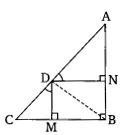# In the given figure, $D$ is a point on hypotenuse $AC$ of $‚àÜABC, DM \perp BC$ and $DN \perp AB$. Prove that:$DN^2 = DM \times AN$"

Given:

$D$ is a point on hypotenuse $AC$ of $∆ABC, DM \perp BC$ and $DN \perp AB$.

To do:

We have to prove that $DN^2 = DM \times AN$

Solution:
Join $BD$.In quadrilateral $MBND$,

$\angle \mathrm{M}+\angle \mathrm{B}+\angle \mathrm{N}+\angle \mathrm{D}=360^{\circ}$

$90^{\circ}+90^{\circ}+90^{\circ}+\angle \mathrm{D}=360^{\circ}$

$\angle \mathrm{D}=360^{\circ}-270^{\circ}$

$=90^{\circ}$

This implies,

Quadrilateral $MBND$ is a rectangle.

Therefore,

$\angle \mathrm{NDB}=\angle \mathrm{MDB}=45^{\circ}$         (Diagonals of a rectangle bisect the angles)

$\angle \mathrm{CDM}=\angle \mathrm{ADN}=45^{\circ}$

In $\triangle \mathrm{CMD}$ and $\triangle \mathrm{BMD}$,

$\angle \mathrm{CMD}=\angle \mathrm{BMD}$

$\angle \mathrm{CDM}=\angle \mathrm{BDM}$

Therefore, by AA similarity,

$\triangle \mathrm{CMD} \sim \triangle \mathrm{BMD}$

This implies,

$\frac{\mathrm{DM}}{\mathrm{MB}}=\frac{\mathrm{MC}}{\mathrm{DM}}$         (By BPT)

$\mathrm{DM}^{2}=\mathrm{MB} \times \mathrm{MC}$

$=\mathrm{DN} \times \mathrm{MC}$      (Since $MB = DN$)

In $\triangle \mathrm{DNB}$ and $\triangle \mathrm{AND}$,

$\angle \mathrm{DMB}=\angle \mathrm{AND}$

$\angle \mathrm{ADN}=\angle \mathrm{BDN}$

Therefore, by AA similarity,

$\triangle \mathrm{DNB} \sim \triangle \mathrm{AND}$

This implies,

$\frac{\mathrm{DN}}{\mathrm{AN}}=\frac{\mathrm{NB}}{\mathrm{DN}}$         (By BPT)

$\mathrm{DN}^{2}=\mathrm{AN} \times \mathrm{NB}$

$\mathrm{DN}^{2}=\mathrm{DM} \times \mathrm{AN}$       (Since $NB = DM$)

Hence proved.